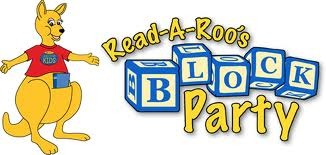# Ruby ruby 的 Block，Proc，Lambda ---2

zputee · 2013年04月24日 · 最后由 u1360749170 回复于 2013年04月24日 · 3138 次阅读一、什么是 ruby 中的代码块？

• 大括号 + 代码 { p 'hi'}
• do ... end，也是代码块
• ruby 中万物皆对象，块不是。

1. proc + 代码块
2. Proc.new + 代码块
3. lambda + 代码块
4. -> + 代码块

``````#一、关于&block的参数
def teach_math(a,b,&block)
puts "let's begin teach math"
block.call(a,b)       #<=
end

#二、关于yield的参数 （与方法一完全等效）
def teach_math2(a,b)
puts "let's begin teach math"
yield(a,b)               #<=
end
#--------------------------------------
#1、最简洁，最常用的
teach_math2(10,2){|x,y| x+y} #加法

#2、Proc实例
pp = Proc.new {|x,y| x-y}    #减法
teach_math2(10,2,&pp)

#3、简写Proc实例
p = proc{|x,y| x-y}
teach_math2(10,2,&p)

#4、lambda
ll= lambda{|x,y| x*y}        # 乘法
teach_math2(10,2,&ll)

#5、简写的lambda
l = ->(x,y){x*y}
teach_math2(10,2,&l)
``````

• 1、3、5 比较简洁。
• lambda 更严谨些。
• lambda，和 Proc.new 有两点细微的差别（1.return，2.参数数量严格否）